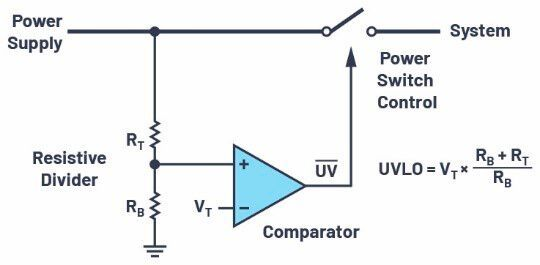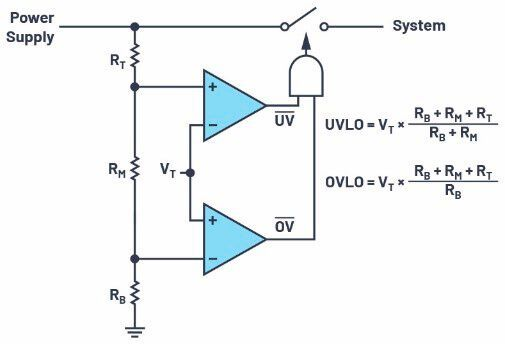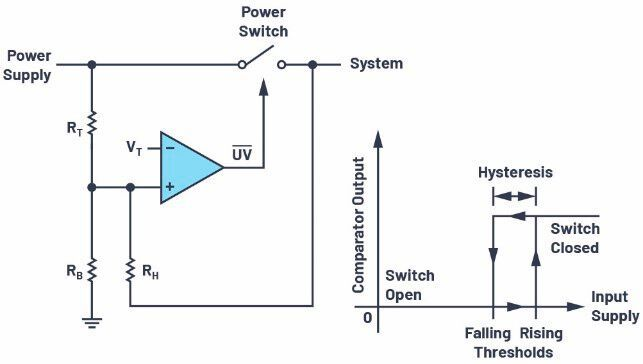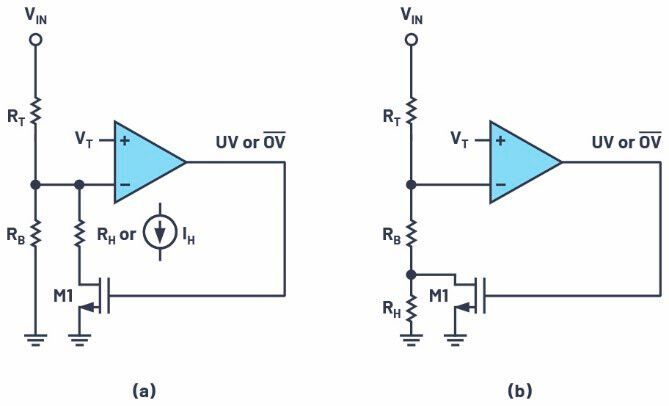## THRESHOLD HYSTERESISAdding hysteresis for smooth undervoltage and overvoltage lockout

From Pinkesh Sachdev

Related Vendorsless

Threshold hysteresis is needed to obtain a smooth and chatter-free lockout function even in the presence of supply noise or resistance. After discussing a simple UVLO/OVLO circuit, this article will present some simple methods for adding threshold hysteresis, which is necessary when the default value is insufficient.Since undervoltage and overvoltage thresholds depend on the system’s valid operating range, resistive dividers are used to set up custom thresholds with the same control circuit. (Source: ©photoschmidt - stock.adobe.com)

Resistive dividers attenuate high voltages down to a level that low voltage circuits can accommodate without getting overdriven or damaged. In power path control circuits, resistive dividers help set up power supply undervoltage and overvoltage lockout thresholds. Such supply voltage qualification circuits are found in automotive systems, battery-powered portable instruments, and data processing and communication boards.

Undervoltage lockout (UVLO) prevents the downstream electronic system from operating with abnormally low power supply voltages, which could cause system malfunction. For example, digital systems can behave erratically or even freeze up when their supply voltage is below specification. When the power supply is a rechargeable battery, undervoltage lockout prevents battery damage due to deep discharge. Overvoltage lockout (OVLO) protects the system from damagingly high supply voltages. Since undervoltage and overvoltage thresholds depend on the system’s valid operating range, resistive dividers are used to set up custom thresholds with the same control circuit. Threshold hysteresis is needed to obtain a smooth and chatter-free lockout function even in the presence of supply noise or resistance.

### Undervoltage and overvoltage lockout circuit

Figure 1 shows an undervoltage lockout circuit (without hysteresis for now). It has a comparator with a positive reference voltage (VT) at its negative input. The comparator controls a power switch that opens or closes the path between the power supply input and the downstream electronic system. The comparator’s positive input connects to a resistive divider from the input. If the supply is turned on and starts rising from 0 V, the comparator output is initially low, keeping the power switch off. The comparator output trips when its positive input reaches VT. At this moment, the current in the bottom resistor is VT/RB. The same current flows in RT if the comparator has no input bias current. Therefore, the supply voltage when the comparator trips is VT + RT × VT/RB = VT × (RB + RT)/RB. This is the supply UVLO threshold set up by the resistive divider. For example, a VT of 1 V and RT = 10 × RT yields a UVLO threshold of 11 V. Below this threshold, the comparator output is low, opening the power switch; above this UVLO threshold, the switch is closed and the supply flows through to power up the system. The threshold can be easily adjusted by changing the ratio of RB and RT. The absolute resistor value is set by the amount of bias current budgeted for the divider (more on this later). To set up an OVLO threshold, just swap the two inputs of the comparator (for example, see the lower comparator in Figure 2) such that a high going input forces the comparator output low and opens the switch.Figure 1. Power supply undervoltage lockout using a resistive divider, comparator, and power switch. (Source: Analog Devices)

While not the focus of this article, the switch can be implemented with an N-channel or a P-channel power MOSFET. The previous discussion assumes an N-channel MOSFET switch that opens (high resistance) when its gate voltage is low (for example, 0 V). To completely close (low resistance) an N-channel MOSFET, the gate voltage must be higher than the supply by at least the MOSFET threshold voltage, requiring a charge pump. Protection controllers such as LTC4365, LTC4367, and LTC4368 integrate comparators and charge pumps to drive N-channel MOSFETs while still consuming low quiescent current. P-channel MOSFETs don’t require a charge pump but the gate voltage polarity is reversed; that is, a low voltage closes whereas a high voltage opens a P-channel MOSFET switch.

Getting back to resistive dividers: a 3-resistor string sets up both undervoltage and overvoltage lockout thresholds (Figure 2), saving one divider’s bias current vs. using two separate 2-resistor strings. The UVLO threshold is VT × (RB + RM + RT)/(RB + RM) while the OVLO threshold is VT × (RB + RM + RT)/RB. An AND gate combines the output of the two comparators before sending it to the power switch. Therefore, the power switch closes to power the system when the input voltage is between the undervoltage and overvoltage thresholds; otherwise, the switch is open, disconnecting the supply from the system. If divider current consumption is not a concern, separate undervoltage and overvoltage dividers provide more flexibility in adjusting each threshold independently of the other.

### Subscribe to the newsletter now

#### Don't Miss out on Our Best Content

Unfold for details of your consentFigure 2. Undervoltage and overvoltage lockout using a single resistive divider. (Source: Analog Devices)

### Undervoltage and overvoltage lockout with hysteresis

In Figure 1, if the power supply rises slowly and has noise or if the supply has inherent resistance (as in a battery) that causes the voltage to drop with load current, the output of the comparator will switch high and low repeatedly as the input crosses its UVLO threshold. This is because the comparator’s positive input repeatedly goes above and below the VT threshold due to the input noise or the drop due to load current through the supply resistance. For battery-powered circuits, this can be a never-ending oscillation. Using a comparator with hysteresis eliminates this chatter, making the switch transition smoother. As illustrated in Figure 3, a hysteretic comparator presents different thresholds for a rising (for example, VT + 100 mV) vs. a falling input (for example, VT – 100 mV). The hysteresis at the comparator level is scaled up by RB and RT to 200 mV × (RB + RT)/RB at the supply level. If the noise or the drop at the supply input is below this hysteresis, the chatter is eliminated. There are ways to add or increase hysteresis if that provided by the comparator is either absent or insufficient. All these methods use positive feedback at the divider tap—for example, a rising comparator input jumps higher when the comparator trips. For simplicity, the following equations assume no intrinsic hysteresis in the comparator.Figure 3. Adding undervoltage lockout threshold hysteresis with a resistor from the divider tap to the power switch output. (Source: Analog Devices)

### Resistor from divider to output (Figure 3):

Add a resistor (RH) from the divider tap (the comparator’s positive input) to the power switch output. When the supply starts rising from 0 V, the comparator’s positive input is below VT and the comparator output is low, keeping the power switch off. Assume that the switch output is at 0 V due to the system load. Hence, RH is in parallel with RB for input threshold calculation. The rising input undervoltage threshold is VT × ((RB || RH) + RT)/(RB || RH), where RB || RH = RB × RH/ (RB + RH). The switch turns on above this threshold, connecting the supply to the system. To calculate the falling input undervoltage threshold, RH is in parallel with RT since the switch is closed, giving the falling input undervoltage threshold as: VT × (RB + (RT || RH))/RB, where RT || RH = RT × RH/(RT + RH). If the comparator itself had some hysteresis, substitute VT with the rising or falling comparator threshold in the previous equations. Recall the Figure 1 example, with VT = 1 V and RT = 10 × RB, where both the rising and falling thresholds are 11 V in the absence of comparator hysteresis or RH. Adding an RH = 100 × RB, as in Figure 3, gives a rising input threshold of 11.1 V and a falling threshold of 10.09 V; that is, a hysteresis of 1.01 V. This method does not work for OVLO because a rising input turns off the power switch, causing RH to pull the comparator input lower (which turns on the switch again) instead of higher.

### Switching in a resistor (Figure 4):

Another method of adding hysteresis is to switch in a resistor that changes the effective value of the bottom resistor. The switched resistor can be in parallel (Figure 4a) or in series (Figure 4b). Consider Figure 4a: when VIN is low—say, 0 V—the comparator’s output (UV or OV node) is high, turning on the N-channel MOSFET M1 and connecting RH in parallel with RB. Assume that M1’s on-resistance is either negligible compared to RH or is included in RH’s value. The rising input threshold is the same as in Figure 3: VT × ((RB || RH) + RT)/(RB || RH). Once VIN is above this threshold, the comparator output is low, turning off M1 and disconnecting RH from the divider. Therefore, the falling input threshold is the same as in Figure 1: VT × (RB + RT)/RB. Continuing our example with VT = 1 V, RT = 10 × RB, and RH = 100 × RB, the rising input threshold is 11.1 V and the falling threshold is 11 V; that is, RH yields a hysteresis of 100 mV. This and the following methods can be used for either undervoltage or overvoltage lockout as their purpose depends on how the comparator output turns on the power switch (not shown).Figure 4. Adding undervoltage or overvoltage lockout threshold hysteresis with a switched (a) shunt resistor or current and (b) a series resistor. (Source: Analog Devices)

The configuration of Figure 4b gives the rising input threshold as VT × (RB + RT)/RB and the falling input threshold as VT × (RB + RH + RT)/(RB + RH). RH = RB/10 in Figure 4, giving 11 V as the rising input threshold and 10.091 V as the falling threshold—that is, 909 mV of hysteresis. This shows that the Figure 4b configuration needs a much smaller RH to yield a much larger hysteresis.

### Switching in a current (Figure 4a):

The resistor RH of Figure 4a can be replaced by a current source IH. This method is used in the LTC4417 and LTC4418 prioritized controllers. When VIN is low, the comparator’s high output enables IH. At the rising input threshold, the negative input of the comparator is at VT. Therefore, the current in RT is IH + VT/RB, yielding the rising threshold as VT + (IH + VT/RB) × RT = VT × (RB + RT)/RB + IH × RT. Once VIN is above this threshold, IH is turned off by the comparator’s low output. Therefore, the falling threshold is the same as in Figure 1: VT × (RB + RT)/RB, and the input threshold hysteresis is IH × RT.

### Resistive divider bias current

The previous equations have assumed that the input bias current of the comparator input is zero while the examples have only considered resistor ratios instead of absolute values. Comparator inputs have both input offset voltage (VOS), reference inaccuracy (which can be clubbed with VOS), and input bias or leakage current (ILK). The zero leakage assumption works out if the divider bias current, VT/RB at Figure 1’s trip point, is much larger than the input leakage. For instance, a divider current that is 100 times the input leakage current keeps leakage-caused input threshold error below 1 %. Another method is to compare the leakage induced threshold error to that from the offset voltage. The comparator nonidealities change the Figure 1 input undervoltage threshold equation to be: (VT ± VOS) × (RB + RT)/RB ± ILK × RT (similar to the previous hysteretic current equation), which can be rewritten as (VT ± VOS ± ILK × RB × RT/(RB + RT)) × (RB + RT)/RB. The input leakage shows up as an error in the comparator’s threshold voltage and this error can be minimized in relation to the offset voltage—that is, ILK × (RB || RT) < VOS, by proper resistor selection.

As an example, the LTC4367 undervoltage and overvoltage protection controller has ±10 nA maximum leakage for the UV and OV pins while the UV/OV pin comparator’s 500 mV threshold offset voltage is ±7.5 mV (±1.5 % of 500 mV). Budgeting a ±3 mV (±0.6 % of 500 mV, or less than half of the 7.5 mV offset) leakage caused threshold error gives RB || RT < 3 mV/10 nA = 300 kΩ. To set up an 11 V input undervoltage threshold with a 0.5 V comparator threshold requires RT = RB × 10.5 V/0.5 V = 21 × RB. Therefore, RB || RT = 21 × RB/22 < 300 kΩ, giving RB < 315.7 kΩ. The nearest 1 % standard value for RB is 309 kΩ, yielding RT to be 6.49 MΩ. The divider bias current at the trip point is 0.5 V/309 kΩ = 1.62 µA, which is 162 times the 10 nA leakage current. This kind of analysis is important when minimizing the divider current without increasing the threshold error due to the comparator’s input leakage current.

### Conclusion

Resistive dividers enable easy adjustment of power supply undervoltage and overvoltage lockout thresholds with the same comparator-based control circuit. Supply noise or resistance requires threshold hysteresis to prevent power switch on and off chattering as the supply crosses the threshold. A few different methods for implementing undervoltage and overvoltage lockout hysteresis have been shown. The essential principle is to have some positive feedback at the divider tap when the comparator trips. When adding or increasing hysteresis of protection controller ICs, some methods depend on the availability of the comparator output or a similar signal at the IC output pins. While picking resistor values, care should be taken that the comparator’s input leakage doesn’t become a dominant source of threshold error. A comprehensive set of related equations, including those in this article, have been implemented in a spreadsheet that’s available to download.